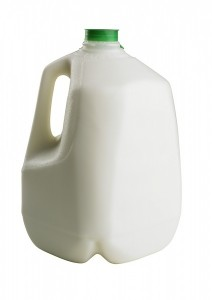# Gallons To Liters

The decision of some countries to go their own way as far as using measurement systems are concerned has produced problems for everyone. This is even more the case particularly if one of those countries that decided to go its on way is the most powerful and influential in the world. Thus, the United States’ decision not to adopt the International System of Units, or the SI, created complications since everyone must now live with a world measured not by meters, Celsius, or grams only but also by feet, Fahrenheit, and pounds.

This makes learning the converting procedures between some of the basic units of measurement from the two systems essential. One such converting procedure that is practical for everyday living is the conversion of gallons to liters. Knowing the conversion factor between gallons and liters is especially useful if one is driving an American-made car that has a gas tank capacity indicated in gallons into a territory that uses the SI’s liters. If one knows how to convert gallons to liters then it would be easy for one to know the equivalent number of liters that one must pack up in one’s car while in that territory.First off, when converting gallons to liters, one must know that gallons are bigger than liters. Hence, the most essential information to have is the number of liters in every gallon. The number is around 3.7854 liters for every 1 gallon.

Now that you have the conversion for the two units, you can now convert from gallons to liters. On this note, it is good to remember this tip: when converting from a bigger unit to smaller one, use multiplication, otherwise division is used. Since we are converting gallons to liters and gallons are bigger units than liters, we use the multiplication function.

Let’s say you drive one of those American-made gas guzzlers that has a fuel tank capacity of 21 gallons. Since you are driving into a place where liters are used you then have to know how much liters you must put in your 21-gallon tank. As was said, when converting gallons to liters, multiplication is used. So we must multiply 21 by the conversion factor, which is 3.7854. 21 X 3.7854 is 79.4934 which is the equivalent number in liters of 21 gallons.

Knowing how to convert from gallons to liters, like other converting operations, is handy especially if you move from place to place that have different systems of measurement in use.